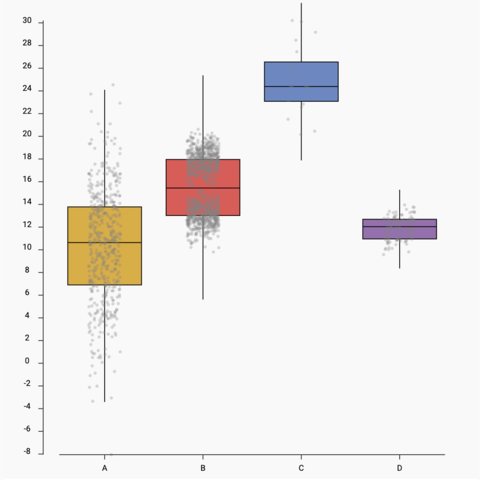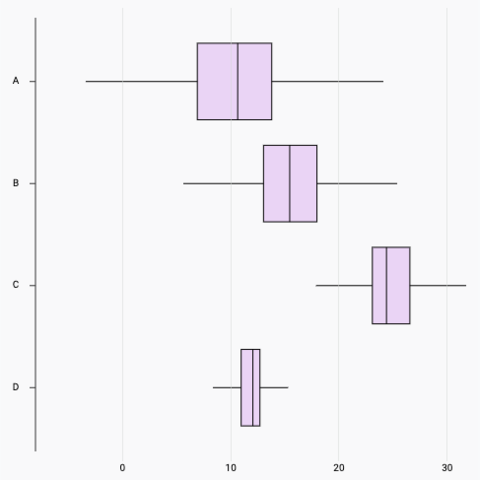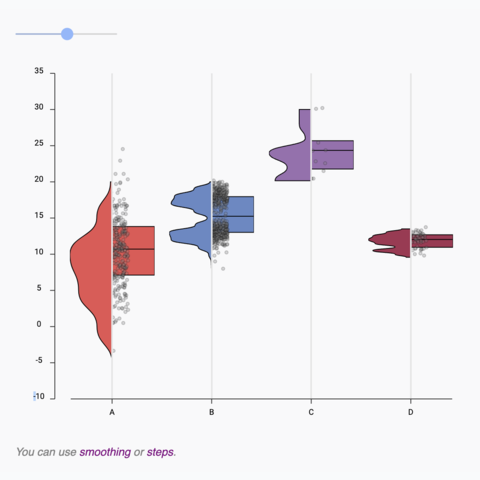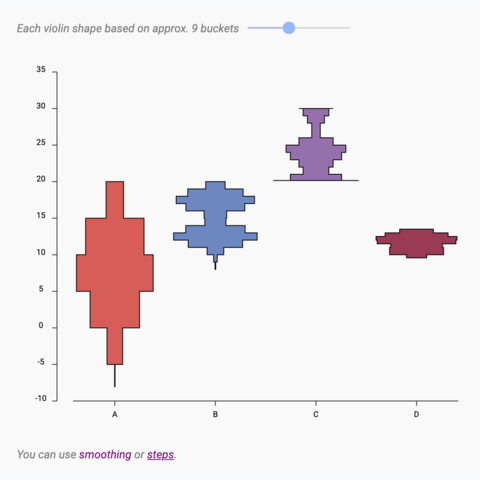# Boxplotwith React and d3.jsA boxplot summarizes the distribution of a numeric variable, often for several groups of a dataset. This page is a step-by-step guide on how to build a reusable boxplot component for the web using React and D3.js.

It starts by describing how to format the dataset and how to initialize the boxplot component. It then explains how to create a `Box` component that displays a single box. Finally, it shows how to render the boxplot and suggests a few variations. 🙇‍♂️.

## The Data 💾

The dataset used to build a boxplot is usually an array of objects. For each object, a `name` property provides the group name, and a `value` property provides the numeric value. It looks like this:

``````const data = [
{ name: "A", value: 10.7577 },
{ name: "A", value: 19.9273 },
{ name: "B", value: 13.8917 },
{ name: "B", value: 0.5102 },
{ name: "C", value: 10.5524 },
...
]``````

## Summary statistics 🔨

A boxplot is based on summary statistics. For a set of values it displays:

• the median: central line of the box
• the first and third quartiles: upper and lower lines of the box
• the min and max values excluding outliers

Let's build a util function called `getSummaryStats` that computes this from an array of numeric values:

``````export const getSummaryStats = (data: number[]) => {
const sortedData = data.sort(function(a, b){return a - b});

const q1 = d3.quantile(sortedData, .25)
const median = d3.quantile(sortedData, .5)
const q3 = d3.quantile(sortedData, .75)

if(!q3 || !q1 || !median){
return
}

const interQuantileRange = q3 - q1
const min = q1 - 1.5 * interQuantileRange
const max = q3 + 1.5 * interQuantileRange

return {min, q1, median, q3, max}
}``````

This function is going to be handy. Now we want to draw a box representing those values.

## A reusable box component 📦

With the output of the `getSummaryStats()` function above we need to draw a box in SVG. Let's create a `VerticalBox` component that does this for us.

There is nothing fancy here. A `rect` is used for the main box. Some `line` are used for the rest.

``````const VerticalBox = ({
min,
q1,
median,
q3,
max,
width,
stroke,
fill,
}) => {
return (
<>
<line
x1={width / 2}
x2={width / 2}
y1={min}
y2={max}
stroke={stroke}
width={STROKE_WIDTH}
/>
<rect
x={0}
y={q3}
width={width}
height={q1 - q3}
stroke={stroke}
fill={fill}
/>
<line
x1={0}
x2={width}
y1={median}
y2={median}
stroke={stroke}
width={STROKE_WIDTH}
/>
</>
);
};``````

This component can be called using the following statement:

``````<svg width={200} height={300}>
<VerticalBox
width={100}
min={280}
q1={200}
median={100}
q3={80}
max={10}
stroke="black"
/>
</svg>``````

Bringing this result:

The `VerticalBox` component allows to draw a vertical box displaying the summary statistics of a set of numeric values

## Component skeleton

The goal here is to create a `Boxplot` component that will be stored in a `Boxplot.tsx` file. This component requires 3 props to render: a `width`, a `height`, and some `data`.

The shape of the `data` is described above. The `width` and `height` will be used to render an `svg` element in the DOM, in which we will insert the histogram.

To put it in a nutshell, that's the skeleton of our `Histogram` component:

``````import * as d3 from "d3"; // we will need d3.js

type BoxplotProps = {
width: number;
height: number;
data: { name: string, value: number }[];
};

export const Boxplot = ({ width, height, data }: BoxplotProps) => {

// compute summary statistics for each group
// compute scales
// build the boxes

return (
<div>
<svg width={width} height={height}>
// render all the boxes
// draw the axes
</svg>
</div>
);
};``````

It's fundamental to understand that with this code organization, d3.js will be used to prepare the SVG `circle`, but it's React that will render them in the `return()` statement. We won't use d3 methods like `append` that you can find in usual d3.js examples.

## Scales and axes

### → Scales

Building a boxplot requires transforming a dimension (e.g. a numeric variable or a group name) in a position in pixels. This is done using a fundamental dataviz concept called scale.

D3.js comes with a handful set of predefined scales.

• `scaleLinear` is what we need for the Y axis. It transforms a numeric value in a position
• ``````const scale = d3.scaleLinear()
.domain([0, 10]) // data goes from 0 to 10
.range([0, 200]); // axis goes from 0 to 200 pixels

scale(0); // 0 -> item with a value of 0 will be at the extreme left of the axis
scale(5); // 100 -> middle of the axis
scale(10); // 200 -> extreme right``````
• `scaleBand` is what we need for the X axis. It transforms a categoric variable (the group `name` here) in a position
• ``````const xScale = useMemo(() => {
return d3
.scaleBand()
.range([0, boundsWidth])
.domain(allXGroups)
}, [data, width]);

// xScale("A") -> 0
// xScale.bandwidth() -> 11``````

To dig more into d3 scales, visit this dedicated page. It's a crucial concept that will be used everywhere in this website.

### → Axes

Axes are rather complicated elements. They are composed of the main segment, several ticks that each have a label, and are often decorated with a title.

Here I suggest creating the axes from scratch and storing them in 2 react components called `AxisBottom` and `AxisLeft`. Those components expect a d3 scale as input and do all the SVG drawings for us.

Compute scales to map numeric values to a 2d canvas. Use custom react components to render axes with react from this scales.

The code for the Y axis component is provided below. The following examples will show how straightforward it is to tweak them to reach other chart styles.

code for the Y axis react component
``````import { useMemo } from "react";
import { ScaleLinear } from "d3";

type AxisLeftProps = {
yScale: ScaleLinear<number, number>;
pixelsPerTick: number;
width: number;
};

const TICK_LENGTH = 10;

export const AxisLeft = ({ yScale, pixelsPerTick, width }: AxisLeftProps) => {
const range = yScale.range();

const ticks = useMemo(() => {
const height = range - range;
const numberOfTicksTarget = Math.floor(height / pixelsPerTick);

return yScale.ticks(numberOfTicksTarget).map((value) => ({
value,
yOffset: yScale(value),
}));
}, [yScale]);

return (
<>
{/* Ticks and labels */}
{ticks.map(({ value, yOffset }) => (
<g
key={value}
transform={"translate(0, {yOffset})"} // TODO struggling with back ticks
shapeRendering={"crispEdges"}
>
<line
x1={-TICK_LENGTH}
x2={width + TICK_LENGTH}
stroke="#D2D7D3"
strokeWidth={0.5}
/>
<text
key={value}
style={{
fontSize: "10px",
textAnchor: "middle",
transform: "translateX(-20px)",
fill: "#D2D7D3",
}}
>
{value}
</text>
</g>
))}
</>
);
};``````

The X axis implementation is very similar. Check the code of the chart below to read it.

## Basic boxplot with React

We now have all the ingredients to cook the final recipe. We have everything to compute the summary statistics for each group of the dataset, and plot the result with several boxes. We also know how to compute scales and add some axes to the chart.

So it is just a matter of looping through all the groups of the dataset and drawing a box for each.

Here is the final result:

Most basic boxplot built with d3.js and React. D3 is used to compute summary statistics and scales. React is used for rendering.

## Responsive Boxplot with react

The component above is not responsive. It expects 2 props called `width` and `height` and will render a Boxplot of those dimensions.

Making the Boxplot responsive requires adding a wrapper component that gets the dimension of the parent `div`, and listening to a potential dimension change. This is possible thanks to a hook called `useDimensions` that will do the job for us.

`useDimensions`: a hook to make your viz responsive
``````export const useDimensions = (targetRef: React.RefObject<HTMLDivElement>) => {

const getDimensions = () => {
return {
width: targetRef.current ? targetRef.current.offsetWidth : 0,
height: targetRef.current ? targetRef.current.offsetHeight : 0
};
};

const [dimensions, setDimensions] = useState(getDimensions);

const handleResize = () => {
setDimensions(getDimensions());
};

useEffect(() => {
return () => window.removeEventListener("resize", handleResize);
}, []);

useLayoutEffect(() => {
handleResize();
}, []);

return dimensions;
}``````

I'm in the process of writing a complete blog post on the topic. Subscribe to the project to know when it's ready.

## Boxplot inspiration

If you're looking for inspiration to create your next Boxplot, note that dataviz-inspiration.com showcases many examples. Definitely the best place to get ... inspiration!

dataviz-inspiration.com showcases hundreds of stunning dataviz projects. Have a look to get some ideas on how to make your Boxplot looks good!

visit

## Boxplot variations

Even if powerful to summarize the distribution of a numeric variable, the boxplot has flaws.

It indeed hides the underlying distribution. For instance, a low sample size or a bi-modal distribution is impossible to detect by reading the boxes only.

Jittering is a good workaround. Add all individual data points with low size, low opacity, and some random shift to the right or the left (jitter). The underlying distribution becomes instantly available.

Note that another good alternative is the violin plot, especially for a high sample size.Boxplot with jitterAdd individual data points using jitter on top of the boxplotHorizontal boxplotHow to build a horizontal boxplot with reactViolin to Boxplot transitionUsing shape morphism to smoothly transition from a boxplot to a violin and reverseViolin with variable bucket sizeA violin plot with a slider to change the bucket size in use

## Contact

👋 Hey, I'm Yan and I'm currently working on this project!

Feedback is welcome ❤️. You can fill an issue on Github, drop me a message on Twitter, or even send me an email pasting `yan.holtz.data` with `gmail.com`. You can also subscribe to the newsletter to know when I publish more content!